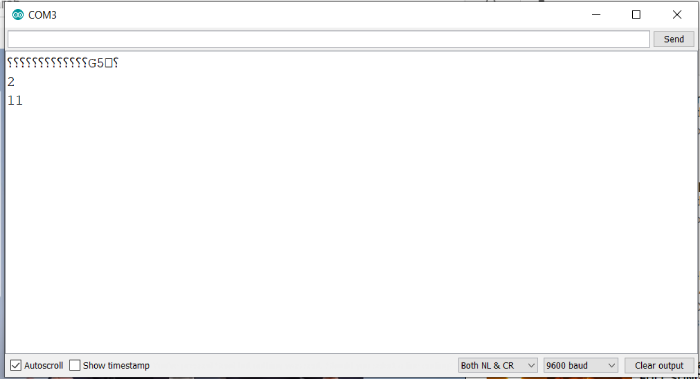# Bitwise AND and OR in Arduino

Bitwise AND/ OR means AND/ OR performed at a bit-level, individually. Each number has its binary representation. When you perform the bitwise AND of one number with another, the AND operation is performed on the corresponding bits of the two numbers. Thus, LSB of number 1 is ANDed with the LSB of number 2, and so on.

The bitwise AND operation in Arduino is & and the bitwise OR operator is |.

## Syntax

a & b

for AND.

a | b

for OR.

The truth table for AND is

PQp & q
000
010
100
111

The truth table for OR is −

PQp & q
000
011
101
111

Since these are bitwise operators, we need to perform this for each bit.

For example, if I have to perform 10 & 3, this is how the operation will look like

 1 0 1 0 10 0 0 1 1 3 0 0 1 0 10 & 3 = 2

As you can see, the AND operator is performed for each of the corresponding bits individually.

Similarly, the operation for 10 | 3 will look like the following −

 1 0 1 0 10 0 0 1 1 3 1 0 1 1 10 | 3 = 2

Please note that this actually applies to all the bits of the number (even the leading 0s). Thus, if your board uses 16-bits to represent an integer, the actual operation (for 10 & 3) will look like −

 0 0 0 0 0 0 0 0 0 0 0 0 1 0 1 0 10 0 0 0 0 0 0 0 0 0 0 0 0 0 0 1 1 3 0 0 0 0 0 0 0 0 0 0 0 0 0 0 0 0 10 & 3 = 2

Same applies for the | operation.

## Example

Let’s verify this through Arduino. The code is given below −

void setup() {
// put your setup code here, to run once:
Serial.begin(9600);
Serial.println();

int a = 10;
int b = 3;
Serial.println(a & b);
Serial.println(a | 3);
}
void loop() {
// put your main code here, to run repeatedly:
}

## Output

The Serial Monitor output is shown below −As you can see, the output is exactly what we expected.